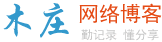# 递归和迭代有什么区别

``````int Find(int *ary,int index,int len,int value)
{
if(len==1)//最后一个元素
{
if (ary[index]==value)return index;//成功查询返回索引
return -1;//失败，返回-1
}
//如果长度大于1，进行折半递归查询
int half=len/2;
//检查被查值是否大于上半部分最后一个值，如果是则递归查询后半部分
if(value>ary[index+half-1])
return Find(ary,index+half,half,value);
//否则递归查询上半部分
return Find(ary,index,half,value);
}``````

``````int v=1;
for(i=2;i<=100;i++)
{
v=v+i;
}``````### 评论

• 欢迎访问木庄网络博客
• 可复制：代码框内的文字。
• 方法：Ctrl+C。